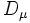# Group-to-representation map

This term is related to: linear representation theory
View other terms related to linear representation theory | View facts related to linear representation theory

## Definition

Let$G$ be a finite group and$k$ a field that is sufficiently large for$G$. A group-to-representation map is an expression of$G$ as a disjoint union:$gtr:G = \bigsqcup_\mu D_\mu \times D_\mu$

where$\mu$ varies over the irreducible representations of$G$, and$D_\mu$ is a set whose size is the degree of$\mu$.

## Properties

We call the group-to-representation map self-adjoint if$gtr(g)$ and$gtr(g^{-1})$ correspond to the same$\mu$ and are simply the same thing with the coordinates flipped.

## Example

The RSK-correspondence gives an example of a group-to-representation map when the group in question is a symmetric group.